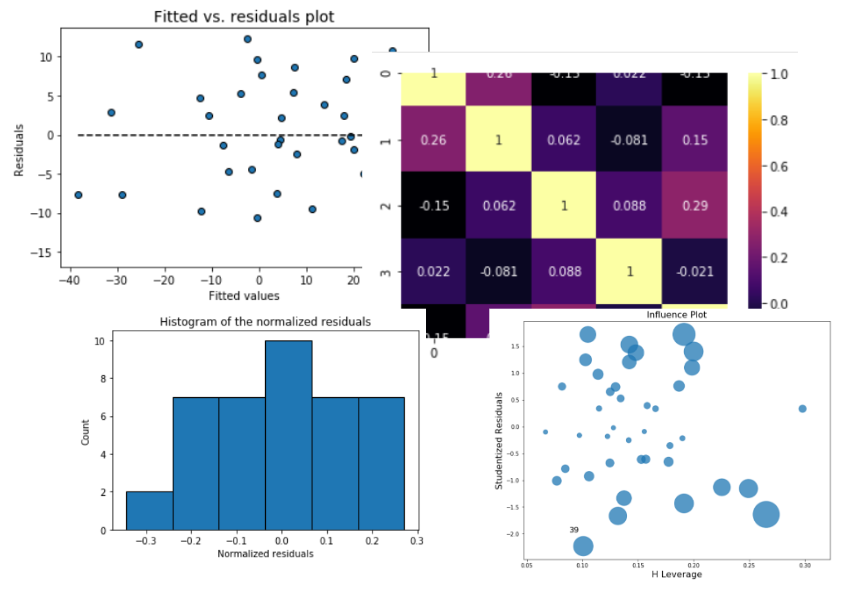# mlr

## What is it?

A lightweight, easy-to-use Python package that combines the `scikit-learn`-like simple API with the power of statistical inference tests, visual residual analysis, outlier visualization, multicollinearity test, found in packages like `statsmodels` and R language.

Authored and maintained by Dr. Tirthajyoti Sarkar (Website, LinkedIn profile)

Here is the Github Repo for the source code.## Features

### Useful regression metrics,

• MSE, SSE, SST
• AIC (Akaike Information Criterion), and BIC (Bayesian Information Criterion)

### Inferential statistics,

• Standard errors
• Confidence intervals
• p-values
• t-test values
• F-statistic

### Visual residual analysis,

• Plots of fitted vs. features,
• Plot of fitted vs. residuals,
• Histogram of standardized residuals
• Q-Q plot of standardized residuals

### Outlier detection

• Influence plot
• Cook's distance plot

### Multicollinearity

• Pairplot
• Variance infletion factors (VIF)
• Covariance matrix
• Correlation matrix
• Correlation matrix heatmap

## Requirements

• numpy (`pip install numpy`)
• pandas (`pip install pandas`)
• matplotlib (`pip install matplotlib`)
• seaborn (`pip install seaborn`)
• scipy (`pip install scipy`)
• statsmodels (`pip install statsmodels`)

## Install

(On Linux and Windows) You can use `pip`

`pip install mlr`

(On Mac OS), first install pip,

``````curl https://bootstrap.pypa.io/get-pip.py -o get-pip.py
python get-pip.py
``````

Then proceed as above.

## Quick Start

Import the `MyLinearRegression` class,

``````from mlr.MLR import MyLinearRegression as mlr
import numpy as np
``````

Generate some random data

``````num_samples=40
num_dim = 5
X = 10*np.random.random(size=(num_samples,num_dim))
coeff = np.array([2,-3.5,1.2,4.1,-2.5])
y = np.dot(coeff,X.T)+10*np.random.randn(num_samples)
``````

Make a model instance,

``````model = mlr()
``````

Ingest the data

``````model.ingest_data(X,y)
``````

Fit,

``````model.fit()
``````